Beginning Factoring MathBitsNotebook.com Terms of Use   Contact Person: Donna Roberts"Factoring is the opposite of multiplying."
When you are asked to "factor" an expression, you are being asked
to write the expression as the product (multiplication) of other expressions.You can think of factoring as playing the game-show "Jeopardy" where you are shown an answer and asked to find the question. In factoring, you are given the answer to a multiplication, and asked to find the numbers or expressions that were multiplied to get this answer. At times, finding factors can be a challenge! But don't panic! The more factoring problems you see, the more strategies you will acquire and the easier "the game" will become. So, get those spidey senses ready.

The first step in every factoring problem is to determine and factor out the greatest common factor.Process: 1. Find the greatest common factor of all terms of the polynomial. 2. To clearly see what is happening, list each term as the product of the GCF and the other factor. 3. Use the distributive property to factor out the GCF.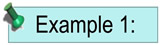Factor:   2x4 + 6x3 - 4x2.

Solution:

1. GCF = 2x2
2. 2x2•x2 + 2x2•3x - 2x2•2
3.
2x2(x2 + 3x - 2) ANSWERYou can always tell if your factoring is correct!! Check your factored answer by multiplying through your answer using the distributive property. The result should be the expression with which you started.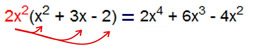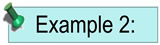Factor:   -10a3 + 5a2 - 15a
Solution:
1. GCF = -5a
2. (-5a)•2a2 + (-5a)•(-a) - (-5a)•(-3)
3. (-5a)(2a2 - a + 3) ANSWERWhen the leading coefficient of a polynomial is negative, it is customary to find a common factor which is negative. (Or think of it as factoring "-1" from each term.)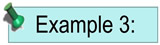Factor:   8m - 64
Solution:
1. GCF = 8
2. 8•m - 8•8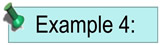Factor:   -20x4 - 50x2
Solution:
1. GCF = -10x2
2. (-10x2)•2x2 - (-10x2)•(-5)
3.

Let's move on to factoring a binomial from a polynomial: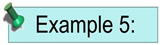Factor:   5x(x + 2) + 9(x + 2)
Solution:
1. GCF = (x + 2)
2. (x + 2)•5x +
(x + 2)•9
3. (x + 2)(5x + 9) ANSWER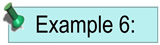Factor:   3a(2a - 1) + 9(2a - 1)
Solution:
1. GCF = 3(2a - 1)
2.
3(2a - 1)•a + 3(2a - 1)•3
3. 3(2a - 1)(a + 3) ANSWERFor help with factoring on your calculator, Click Here!